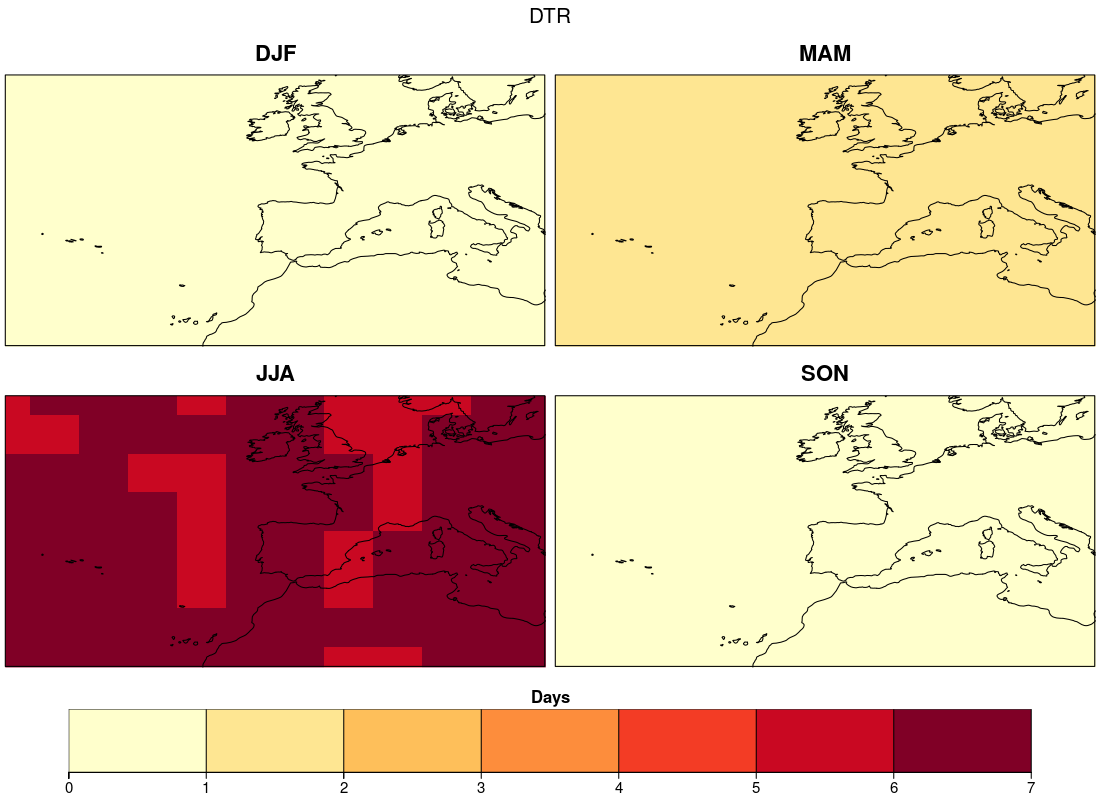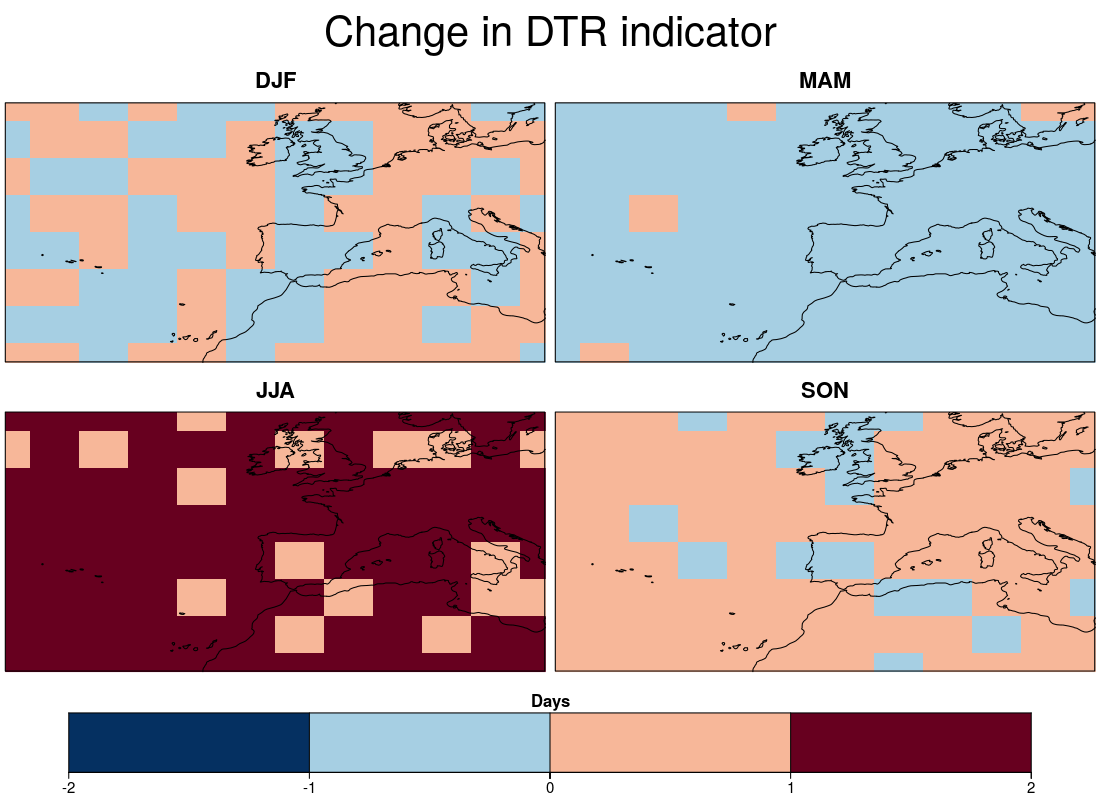# Diurnal Temperature Variation (DTR) Indicator

The diurnal temperature variation indicator is a proxy for energy demand. The diurnal temperature indicator is the number of days in a season when the daily temperature variation (tasmax - tasmin) exceeds the vulnerability threshold. Here, the vulnerability threshold is based on the mean daily temperature variation for a reference period plus 5 degrees.

### 1- Load dependencies

This example requires the following system libraries:

• libssl-dev
• libnecdf-dev
• cdo

The ClimProjDiags R package should be loaded by running the following lines in R, once it is integrated into CRAN mirror.

``````library(ClimProjDiags)
``````

All the other R packages involved can be installed directly from CRAN and loaded as follows:

``````library(s2dv)
library(abind)
library(parallel)
``````

### 2- Problem, parameters and data definition

To ilustrate the problem, daily maximum or minimum air temperature at 2 m for the reference period (1971 - 2000) and the future scenario rcp2.6 (2006 - 2100) in the northern hemisphere (between -40 - 20 ºE and 25 - 60 ºN) will be created artificially.

A grid of 5 degrees is defined by running the next lines in R:

``````lat <- seq(25, 60, 5)
lon <- seq(-35, 20 ,5)
``````

The synthetic sample of maximum and minimum temperature for the historical period can be obtained by running the following lines. The maximum temperature data is built by adding random perturbation to a sinusoidal function. The latitudinal behavior of the temperature is computed by randomly subtracting a value proportional to the latitude. The minimum temperature is built by subtracting a random value (with anual cycle included) to the synthetic maximum temperature. Furthermore, attributes of time and dimensions are added to both samples.

``````tmax_historical <- NULL
tmin_historical <- NULL
grid1 <- 293 - 10 * cos(2 * pi / 365 * (1 : 10958)) + rnorm(10958)
grid2 <- 289 - 8 * cos(2 * pi / 365 * (1 : 10958)) - abs(rnorm(10958))

gridlon1 <- NULL
gridlon2 <- NULL
for (i in 1 : 12) {
gridlon1 <- cbind(gridlon1,
grid1 + abs(rnorm(10958, mean = 2, sd = 1) *
cos(2 * pi / 365 * (1 : 10958))))
gridlon2 <- cbind(gridlon2,
grid2 - abs(rnorm(10958, mean = 2, sd = 1) *
cos(2 * pi / 365 * (1 : 10958))))
}

for (j in 1 : 8) {
gridnew1 <- apply(gridlon1, 2, function(x) {x - rnorm(10958, mean = j * 0.001,
sd = 0.1)})
gridnew2 <- apply(gridlon2, 2, function(x) {x -
abs(rnorm(10958, mean = j * 0.75, sd = 0.5))})
tmax_historical <- abind(tmax_historical, gridnew1, along = 3)
tmin_historical <- abind(tmin_historical, gridnew2, along = 3)
}

names(dim(tmax_historical)) <- c('time', 'lon', 'lat')
names(dim(tmin_historical)) <- c('time', 'lon', 'lat')

tmax_historical <- InsertDim(InsertDim(tmax_historical, posdim = 1,
lendim = 1, name = 'var'),
posdim = 1, lendim = 1, name = 'model')
tmin_historical <- InsertDim(InsertDim(tmin_historical, posdim = 1,
lendim = 1, name = 'var'),
posdim = 1, lendim = 1, name = 'model')

time <- seq(ISOdate(1971, 1, 1), ISOdate(2000, 12, 31), "day")
metadata <- list(time = list(standard_name = 'time', long_name = 'time',
calendar = 'proleptic_gregorian',
units = 'days since 1970-01-01 00:00:00', prec = 'double',
dim = list(list(name = 'time', unlim = FALSE))))
attr(time, "variables") <- metadata
attr(tmax_historical, 'Variables')\$dat1\$time <- time
attr(tmin_historical, 'Variables')\$dat1\$time <- time
``````

A similar procedure is done to build the synthetic data for the future projections. However, a trend is added.

``````tmax_projection <- NULL
tmin_projection <- NULL
grid1 <- 293 - 10 * cos(2 * pi / 365 * (1 : 34698)) + rnorm(34698) +
(1 : 34698) * rnorm(1, mean = 4) / 34698

grid2 <- 289 - 8 * cos(2 * pi / 365 * (1 : 34698)) - abs(rnorm(34698)) +
(1 : 34698) * rnorm(1, mean = 4) / 40000
gridlon1 <- NULL
gridlon2 <- NULL
for (i in 1 : 12) {
gridlon1 <- cbind(gridlon1,
grid1 + abs(rnorm(34698, mean = 2, sd = 1) *
cos(2 * pi / 365 * (1 : 34698))))
gridlon2 <- cbind(gridlon2,
grid2 - abs(rnorm(34698, mean = 2, sd = 1) *
cos(2 * pi / 365 * (1 : 34698))))
}

for (j in 1 : 8) {
gridnew1 <- apply(gridlon1, 2, function(x) {x - rnorm(34698, mean = j * 0.01,
sd = 0.1)})
gridnew2 <- apply(gridlon2, 2, function(x) {x -
abs(rnorm(34698, mean = j * 0.75, sd = 0.5))})
tmax_projection <- abind(tmax_projection, gridnew1, along = 3)
tmin_projection <- abind(tmin_projection, gridnew2, along = 3)
}

names(dim(tmax_projection)) <- c("time", "lon", "lat")
names(dim(tmin_projection)) <- c("time", "lon", "lat")

tmax_projection <- InsertDim(InsertDim(tmax_projection, posdim = 1,
lendim = 1, name = 'var'),
posdim = 1, lendim = 1, name = 'model')
tmin_projection <- InsertDim(InsertDim(tmin_projection, posdim = 1,
lendim = 1, name = 'var'),
posdim = 1, lendim = 1, name = 'model')

time <- seq(ISOdate(2006, 1, 1), ISOdate(2100, 12, 31), "day")
metadata <- list(time = list(standard_name = 'time', long_name = 'time',
calendar = 'proleptic_gregorian',
units = 'days since 1970-01-01 00:00:00', prec = 'double',
dim = list(list(name = 'time', unlim = FALSE))))
attr(time, "variables") <- metadata
attr(tmax_projection, 'Variables')\$dat1\$time <- time
attr(tmin_projection, 'Variables')\$dat1\$time <- time
``````

### 3- Reference diurnal temperature variation

The function `DTRRef`, from the ClimProjDiags package, computes the mean between maximum and minimum temperature for each season during the selected reference period:

``````dtr_reference <- DTRRef(tmax = tmax_historical, tmin = tmin_historical, by.seasons = TRUE, ncores = NULL)
``````

The label `dtr_reference` contains the output for each season and gridpoint:

``````> str(dtr_reference)
List of 2
\$ dtr.ref: num [1:4, 1, 1, 1:12, 1:8] 5.09 2.9 5.04 2.91 5.09 ...
\$ season : chr [1:4] "DJF" "MAM" "JJA" "SON"
``````

### 4- Computing the diurnal temperature variation indicator

The diurnal temperature variation indicator is computed with the function `DTRIndicator` indicating the maximum and minimum temperature for the future projection and the reference temperature variation:

``````dtr_indicator <- DTRIndicator(tmax = tmax_projection, tmin = tmin_projection,
ref = dtr_reference,
by.seasons = TRUE, ncores = NULL)
``````

The function returns a list of three elements, the label `indicator` being the desired output:

``````> str(dtr_indicator)
List of 3
\$ indicator: int [1:96, 1:4, 1, 1, 1:12, 1:8] 0 1 0 0 0 2 0 1 1 0 ...
\$ year     : chr [1:96] "2006" "2007" "2008" "2009" ...
\$ season   : chr [1:4] "DJF" "JJA" "MAM" "SON"
``````

Note: the total number of years in `dtr_indicator\$year` (96) is greater than the period examined (in this case 95 years of future projection). This is because the last december of the time series belongs to the subsequent winter (in this example 2101 winter).

### 5- Visualizing the diurnal temperature variation indicator

A four panel plot can be generated to visualize the seasonal indicator by running the following lines:

``````dtr_rcp <- array(dim = c(length(lon), length(lat), 4))
for (j in 1:4){
dtr_rcp[,,j] <- MeanDims(dtr_indicator\$indicator[,j,,,,], 'year')
}
breaks <- 0 : (max(dtr_rcp) + 1)

PlotLayout(PlotEquiMap, c(1, 2), lon = lon, lat = lat, var = dtr_rcp,
titles = c('DJF', 'MAM', 'JJA', 'SON'),
toptitle = "DTR", filled.continents = FALSE,
units = "Days", title_scale = 0.5, axelab = FALSE,
draw_separators = TRUE, subsampleg = 1,
brks = breaks, color_fun = clim.palette("yellowred"),
bar_extra_labels = c(2, 0, 0, 0),
fileout = "SpatialDTR.png")
``````Furthermore, the future diurnal temperature variation can be compared with the one observed during the reference period. So, the diurnal temperature variation indicator is computed for the reference period by running:

``````dtr_indicator_reference <- DTRIndicator(tmax = tmax_historical,
tmin = tmin_historical,
ref = dtr_reference, by.seasons = TRUE,
ncores = NULL)
``````

The comparison between the reference and the future projection diurnal temperature variation indicator can be computed With a simple subtraction. To visualize the result, `PlotLayout` function will be applied again. The resulting plot will be saved in the working directory.

``````dtr_diff <- array(dim = c(length(lat), length(lon), 4))
for (i in 1:4){
dtr_diff[,,i] <- MeanDims(dtr_indicator\$indicator[, i, 1, 1, , ], 'year') -
MeanDims(dtr_indicator_reference\$indicator[, i, 1, 1, , ], 'year')
}

PlotLayout(PlotEquiMap, c(1, 2), lon = lon, lat = lat, var = dtr_diff,
titles = c('DJF', 'MAM', 'JJA', 'SON'),
toptitle = "Change in DTR indicator",
filled.continents = FALSE, units = "Days",
axelab = FALSE, draw_separators = TRUE, subsampleg = 1,
brks = -2 : 2, bar_extra_labels = c(2, 0, 0, 0),
fileout = "SpatialComparisonDTR.png")
``````Note: the outputs of this example, including the figures, are artificial.